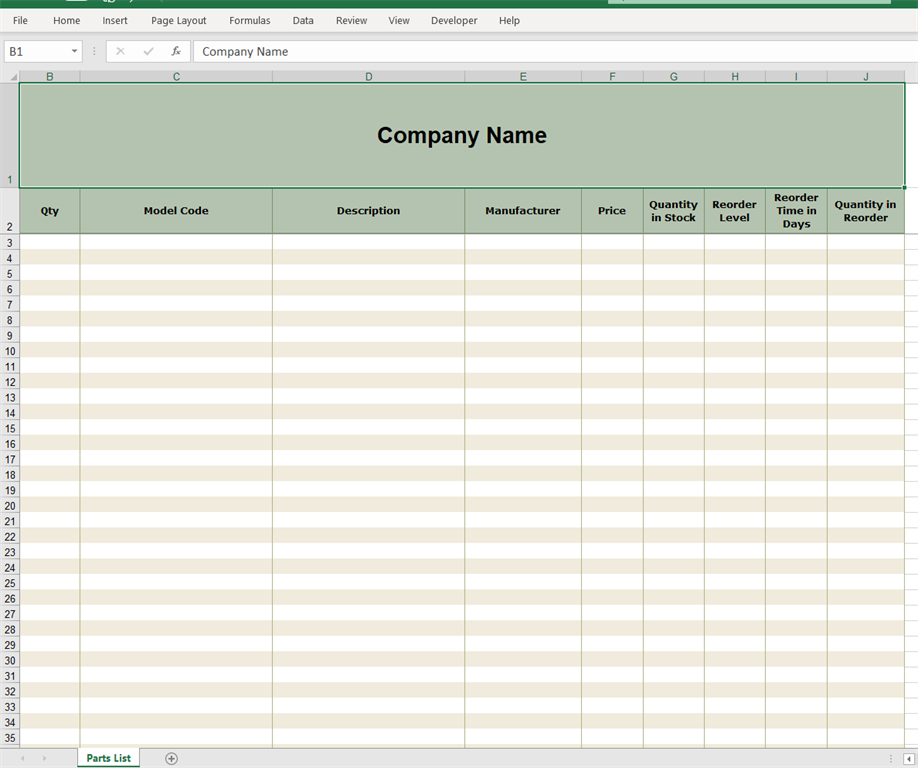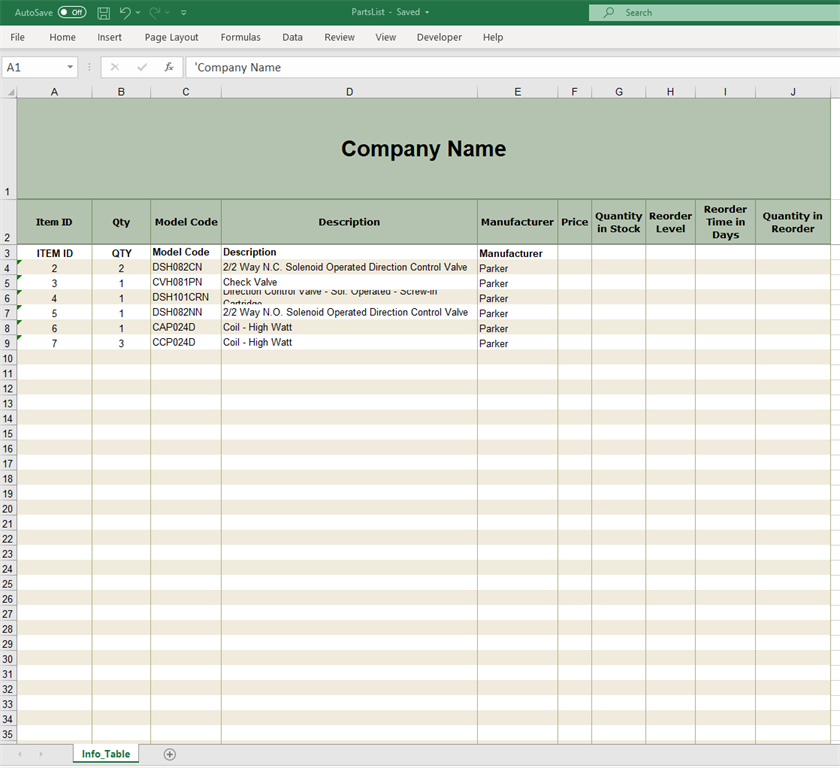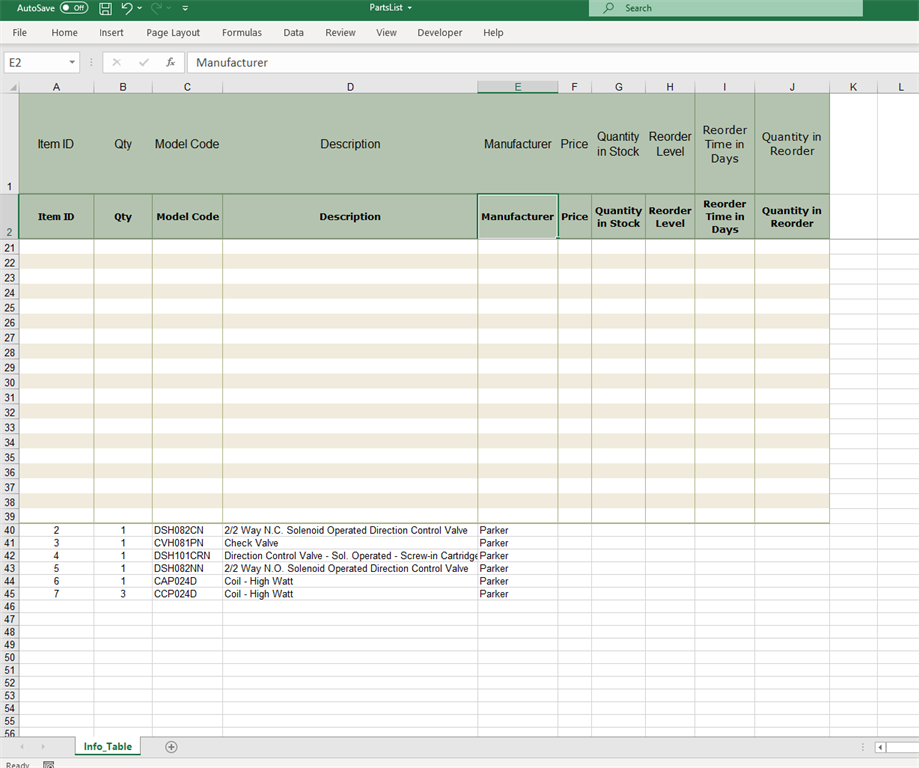# Start exporting data to an excel file from a particular row of sheet

Mar 25 2021 1:42 PMHi everyone,

I have excel installed in my system which is used by my project and I am trying to export the data to an excel file which has a template like this:I want the data to be inserted from the 3rd row without affecting first two rows same as hereBut in the actual output first row is being overwritten by column names and data is inserted from where the template ends :Here is the code by which I was inserting the data :
1. ''' <summary>
2. ''' Export datagridview's data contained in an data table to excel file
3. ''' </summary>
4. ''' <param name="dataTable">DataGridView's datatable</param>
5. ''' <param name="XLPath"> Excel File Path with xlsx extension</param>
6.
7.  Private Shared Sub ExportToExcel(ByVal dataTable As DataTable, ByVal XLPath As String)
8.  Dim connStr = "Provider = Microsoft.ACE.OLEDB.12.0;Data Source=" + XLPath + ";Extended Properties='Excel 8.0;HDR = YES';"
9.  Using connection As OleDbConnection = New OleDbConnection(connStr)
10.       connection.Open()
11.     Using command As OleDbCommand = New OleDbCommand()
12.        command.Connection = connection
13.        Dim columnNames As New List(Of String)
14.        Dim tableName As String = dataTable.TableName
15.        If dataTable.Columns.Count <> 0 Then
16.           For Each dataColumn As DataColumn In dataTable.Columns
18.           Next
19.        Else
20.           tableName = If(Not String.IsNullOrWhiteSpace(dataTable.TableName), dataTable.TableName, Guid.NewGuid().ToString()) + "\$"
21.           command.CommandText = \$"CREATE TABLE [{tableName}] ({String.Join(",", columnNames.[Select](Function(c) \$"[{c}]
22.                                VARCHAR").ToArray())});"
23.           command.ExecuteNonQuery()
24.        End If
25.        If dataTable.Rows.Count <> 0 Then
26.           For Each row As DataRow In dataTable.Rows
27.              Dim rowValues As List(Of String) = New List(Of String)()
28.              For Each column As DataColumn In dataTable.Columns
29.                 rowValues.Add(If((row(column) IsNot Nothing AndAlso Not row(column).Equals(DBNull.Value)),
30.                                   row(column).ToString(), String.Empty))
31.              Next
32.              command.CommandText = \$"INSERT INTO [{tableName}]({String.Join(",", columnNames.[Select](Function(c) \$"[{c}]"))})
33.                            VALUES ({String.Join(",", rowValues.[Select](Function(r) \$"'{r}'").ToArray())});"
34.              command.ExecuteNonQuery()
35.           Next
36.        End If
37.     End Using
38.   End Using
39.  End Sub